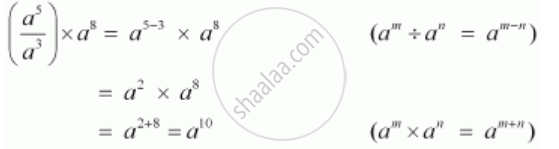Share

# Simplify and Express the Following in Exponential Form (Aexponent5/Aexponent3) Xx Aexponent8 - CBSE Class 7 - Mathematics

ConceptMiscellaneous Examples Using the Laws of Exponents

#### Question

Simplify and express the following in exponential form

(a^5/a^3)  xx a^8

#### SolutionIs there an error in this question or solution?

#### APPEARS IN

NCERT Solution for Mathematics for Class 7 (2018 to Current)
Chapter 13: Exponents and Powers
Ex. 13.20 | Q: 2.1 | Page no. 260
Solution Simplify and Express the Following in Exponential Form (Aexponent5/Aexponent3) Xx Aexponent8 Concept: Miscellaneous Examples Using the Laws of Exponents.
S